# dec dec dec

Author: trupples
Contest: TokyoWesterns CTF 4th 2018

## Proof of Flag

``````TWCTF{base64_rot13_uu}
``````

## Summary

The program base64encodes input, rot13 that, then base64encodes the rot13 result using an unusual alphabet: characters 32 (space) to 96 (backtick). At the end it checks if the result equals `@25-Q44E233=,>E-M34=,,\$LS5VEQ45)M2S-),7-\$/3T `.

## Proof of solving

I opened the binary in IDA and located the main function: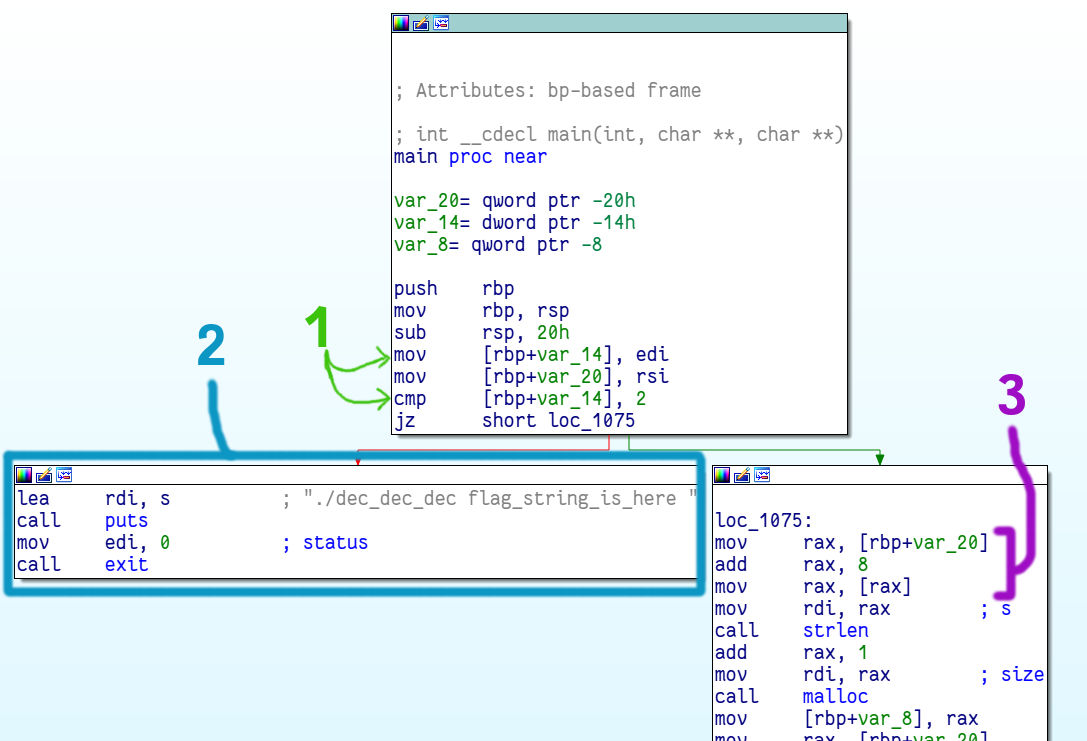The left branch (blue #2) prints some usage information and is being jumped to if `var_14` is not equal to 2 (green #1). It’s safe to assume then that `var_14` is `argc`.

The purple #3 instructions load the value in `var_20`, add 8 to it and use that as a pointer. Then it passes that pointer to `strlen`, so it should be a string. `var_20` is the `argv` array of string pointers to the command line arguments. 8 is added to the array address to access the second element because each element is a memory address and on 64bit addresses take 8 bytes.

### main()

Let’s take a look at the next portion of main: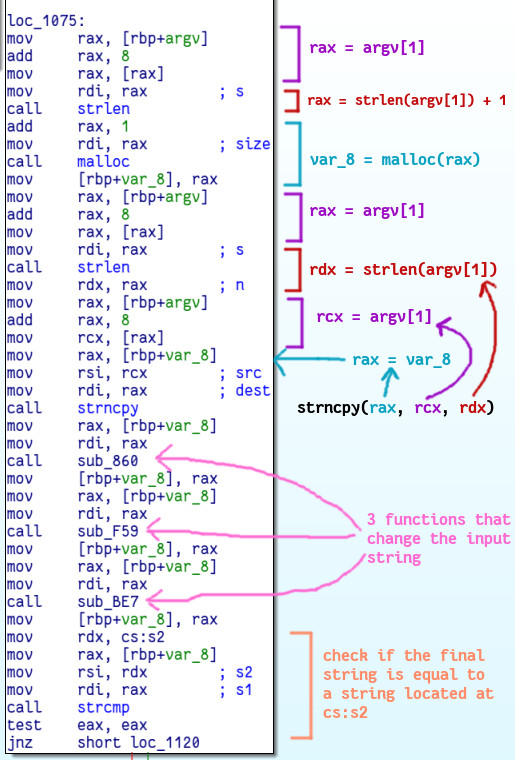The first three instructions are the ones I talked about in the last part. They load `argv` into `rax`. After that `strlen` is called to get the length of the argument, then that is incremented and passed to `malloc` to generate a region of memory that will fit a copy of the string (plus one for the null terminator). Then we call strlen again to recalculate the length of argv (it hasn’t changed; this is redundant) and use `strncpy` to copy that many bytes from the argument to this new memory region.

After the `strncpy` call the copied string is passed through three functions that change it. In the end the modified string will be compared with a string at address `cs:s2`. That final correct string is:

`@25-Q44E233=,>E-M34=,,\$LS5VEQ45)M2S-),7-\$/3T `

### First function

Let’s take a look at the first function! It’s pretty long, but we don’t really need to analyse at all of it. We can just take a brief look. Here are some important snippets. The first looks like the hex encoding of some ASCII letters, so I converted them to characters: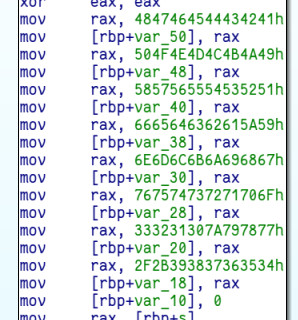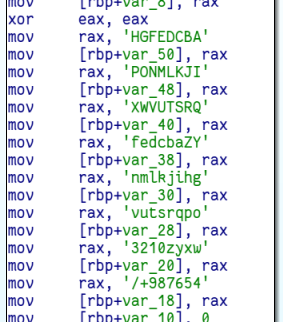The array this is initialising looks like the usual base64 alphabet. If we look further we can see two branches - one which adds an equal sign to some string and one which adds two equal signs to that string. That’s just like the standard base64 padding: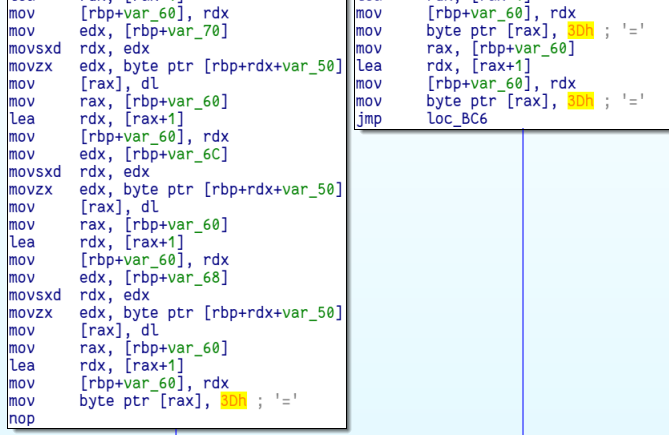The last important thing to notice in this function is that at the beginning it `malloc()`s the destination string and puts the returned address in `var_58` and then at the end it returns that address (through the `rax` register):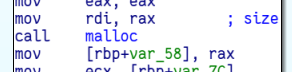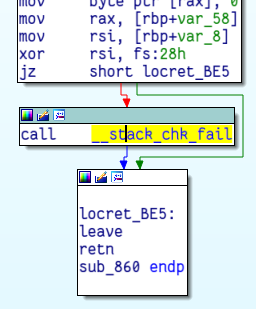### Second functions

This one is considerably shorter. It also `malloc()`s a new buffer and returns it. Then it loops through the input string. For each character, if it’s not a letter it just writes it as-is to the new buffer. For letters there are two branches that handle lowercase and uppercase.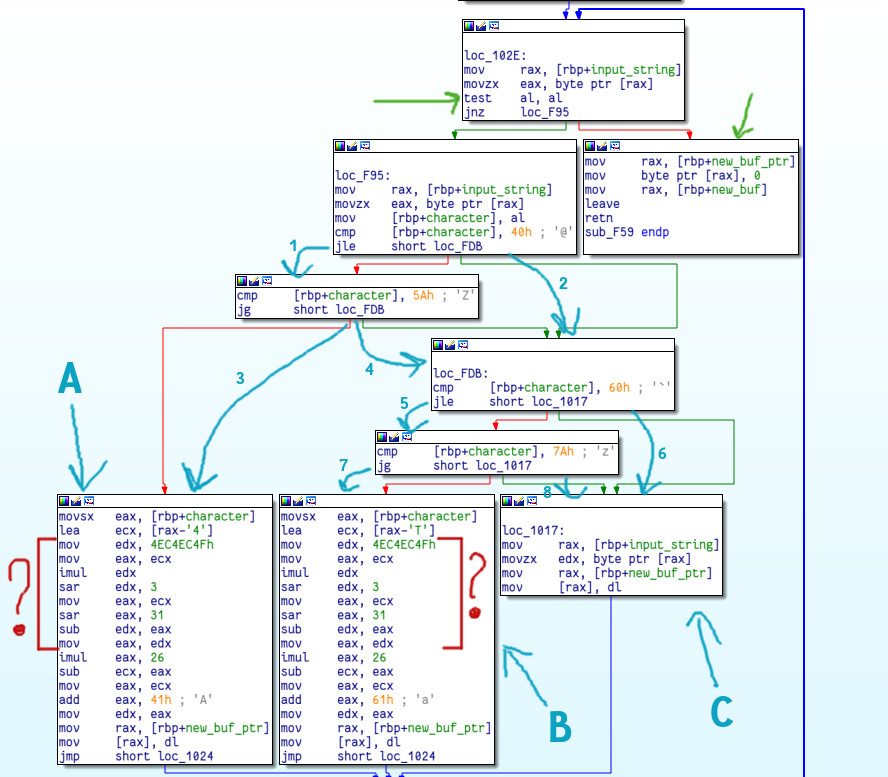The green arrows show the fetching of a character from the input string.

The execution follows blue arrow 1 if the character is greater than 0x40 = 64 = ‘A’-1. After that if it’s also less than or equal to 0x5A = 90 = ‘Z’ arrow 3 is followed and we end up in block A (uppercase letters). However, if either arrow 1 or arrow 3 isn’t taken then arrow 2 or arrow 4 will be followed. We know that the current character is not an uppercase letter. If it’s greater than 0x60 = ‘a’-1 (arrow 5) and less than 0x7a = ‘z’ (arrow 7) we enter block B which handles lowercase letters. If we don’t take that route we’ll end up following either arrow 6 or 8 and that takes us to block C which handles non-letter characters.

The easiest one to understand is block C which just copies the source character unmodified.

The other two blocks do some weird arithmetic (red question marks) on the input character. They first subtract ‘4’ or ‘T’, which basically gets the alphabet index of the character plus 13. I didn’t understand the operations so I searched for the 0x4ec4ec4f constant and found this:

http://www.flounder.com/multiplicative_inverse.htm

It seems like “the mystery code” is dividing the value by 26, then multiplying the rounded down result by 26 and subtracting that from the initial value. That’s an interesting way to calculate the number modulo 26.

Hmmmm… it first calculates the index of the character in the alphabet plus 13, then calculates that mod 26, then puts the corresponding letter in the output string. That’s ROT13!

Now that we’ve figured out the first function is base64 encoding and the second one is ROT13, let’s take a look at the last:

### The third function

This one took a while to figure what it does and I’m not going to go into a huge amount of detail here, unfortunately :( (I’m running out of time).

I recognized this was an algorithm similar to base64 firstly because of the `malloc` size. It allocates `initial_size*4/3` bytes and that’s typical of base64.

Another reason is the usage of the bitwise operations which are commonly also used in base64 encoding. If we have an input block `char A` which will be encoded to the output block `char B`, the following operations will be done:

``````/* (1) */ B = alphabet[A >> 2];
/* (2) */ B = alphabet[((A << 6) & 0x30) | (A >> 4)];
/* (3) */ B = alphabet[((A << 4) & 0x3C) | (A >> 6)];
/* (4) */ B = alphabet[A & 63];
``````

We can see quite similar operations inside the function: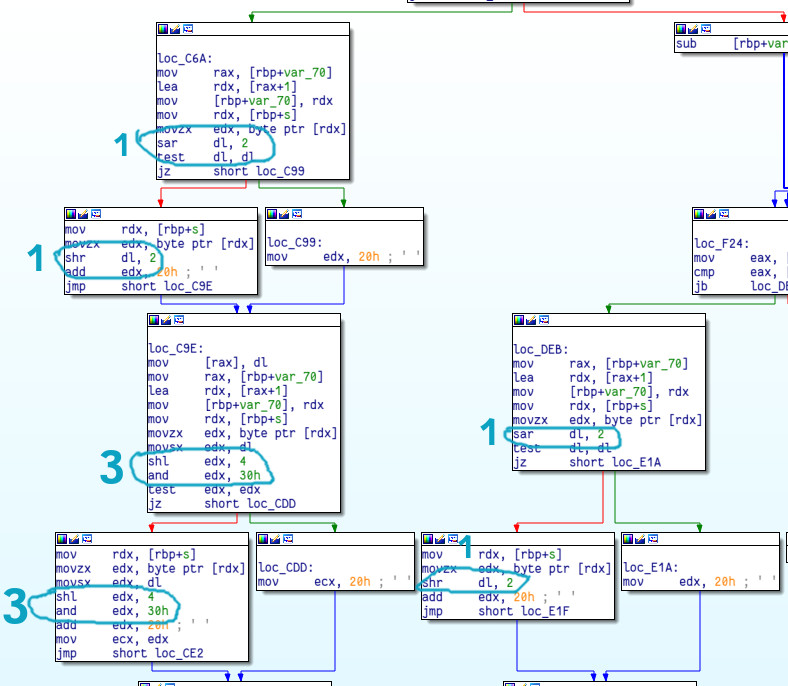So indeed it seems like base64 encryption but instead of using the standard “ABCD..XYZabc…xyz0123..89/+” alphabet it uses characters starting at ascii index 0x20 - the space character.

One annoying thing it does is prepending an @ character and if we don’t look out for that it won’t decode properly. I’ll just ignore that character.

### Reversing the final string

``````targetString = "@25-Q44E233=,>E-M34=,,\$LS5VEQ45)M2S-),7-\$/3T "
``````

The first thing to do would be to base64 decode using the special alphabet:

``````decodedBits = ""
for c in targetString[1:]:
decodedBits += bin(ord(c) - ord(' ') + 64)[-6:]
decodedText = ""
for i in range(0, len(decodedBits), 8):
decodedText += chr(int(decodedBits[i:i+8], 2))
print(decodedText) # => ISqQIRM7LzSmMGL0K3WiqQRmK3I1sD==
``````

Now let’s ROT13 that:

``````rot13edText = ""
for c in decodedText:
if ord(c) >= ord('a') and ord(c) <= ord('z'):
rot13edText += chr((ord(c) - ord('a') + 13) % 26 + ord('a'))
elif ord(c) >= ord('A') and ord(c) <= ord('Z'):
rot13edText += chr((ord(c) - ord('A') + 13) % 26 + ord('A'))
else:
rot13edText += c
print(rot13edText) # => IFdQIRM7LmFzMGL0K3JvdQRzK3I1fD==
``````

And finally, let’s base64 decode that:

``````from base64 import b64decode
print(b64decode(rot13edText))    # => TWCTF{base64_rot13_uu}
``````